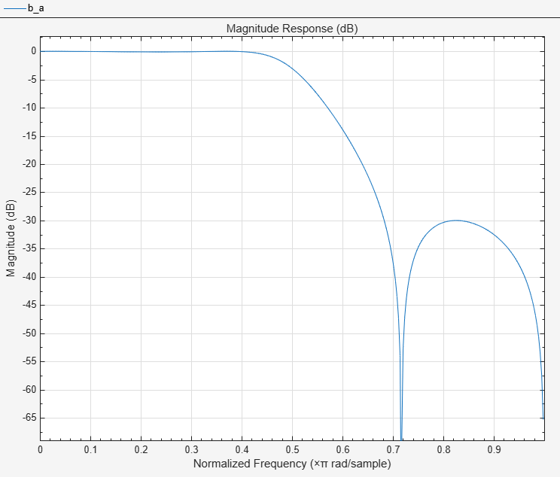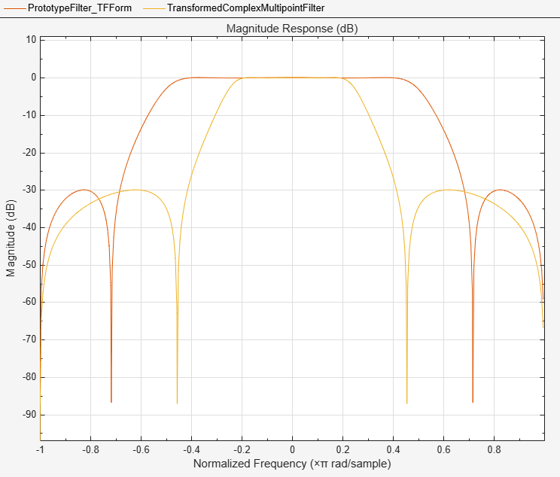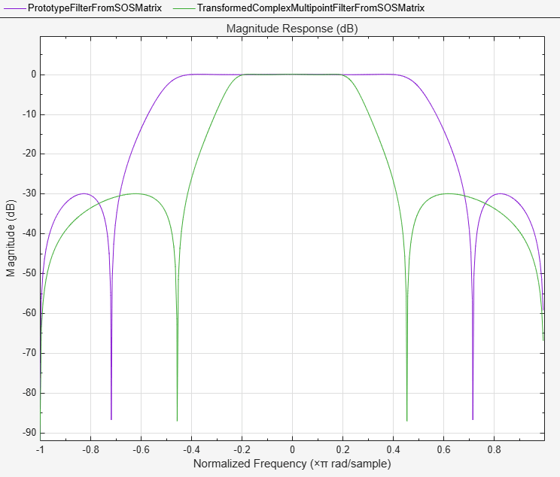# iirlp2xc

Transform IIR lowpass filter to IIR complex N-point filter

## Syntax

``````[num,den,allpassNum,allpassDen] = iirlp2xc(b,a,wo,wt)``````

## Description

example

``````[num,den,allpassNum,allpassDen] = iirlp2xc(b,a,wo,wt)``` transforms an IIR lowpass filter to an IIR complex N-point filter.The prototype lowpass filter is specified with the numerator `b` and the denominator `a`. The function returns the numerator and the denominator coefficients of the transformed IIR complex N-point filter. The function also returns the numerator, `allpassNum`, and the denominator, `allpassDen`, of the allpass mapping filter. The function transforms a real lowpass prototype filter to an N-point complex filter by applying an Nth-order real lowpass to complex multipoint frequency transformation, where N is the number of features being mapped and is given by the length of the vector `wo`. For more details, see IIR Lowpass Filter to IIR Complex N-Point Filter Transformation.```

## Examples

collapse all

Transform a lowpass IIR filter to an N-point IIR complex filter using the `iirlp2xc` function.

Input Lowpass IIR Filter

Design a prototype real IIR lowpass elliptic filter with a gain of about –3 dB at 0.5π rad/sample.

```[b,a] = ellip(3,0.1,30,0.409); fvtool(b,a)```Transform Filter Using `iirlp2xc`

Transform the real prototype lowpass filter to an IIR complex N-point filter.

Specify the prototype filter as a vector of numerator and denominator coefficients, `b` and `a` respectively.

`[num,den] = iirlp2xc(b,a,[-0.5 0.5],[-0.25 0.25]);`

Compare the magnitude response of the filters using FVTool. The transformed filter has complex coefficients and is indeed a bandpass filter.

```fvt = fvtool(b,a,num,den); legend(fvt,"Prototype Filter (TF Form)",... "Transformed Complex Multipoint Filter")```Alternatively, you can also specify the input lowpass IIR filter as a matrix of coefficients. Pass the second-order section matrices as inputs. The numerator and the denominator coefficients of the transformed filter are given by `num2` and `den2`, respectively.

```ss = tf2sos(b,a); [num2,den2] = iirlp2xc(ss(:,1:3),ss(:,4:6),... [-0.5 0.5],[-0.25 0.25]);```

Compare the magnitude response of the filters using FVTool.

```hvft = fvtool(ss,[num2 den2]); legend(hvft,"Prototype Filter (SOS Form)",... "Transformed Complex Multipoint Filter")```## Input Arguments

collapse all

Numerator coefficients of the prototype lowpass IIR filter, specified as either:

• Row vector –– Specifies the values of [b0, b1, …, bn], given this transfer function form:

`$H\left(z\right)=\frac{B\left(z\right)}{A\left(z\right)}=\frac{{b}_{0}+{b}_{1}{z}^{-1}+\cdots +{b}_{n}{z}^{-n}}{{a}_{0}+{a}_{1}{z}^{-1}+\cdots +{a}_{n}{z}^{-n}},$`

where n is the order of the filter.

• Matrix –– Specifies the numerator coefficients in the form of an P-by-(Q+1) matrix, where P is the number of filter sections and Q is the order of each filter section. If Q = 2, the filter is a second-order section filter. For higher-order sections, make Q > 2.

`$b=\left[\begin{array}{ccccc}{b}_{01}& {b}_{11}& {b}_{21}& ...& {b}_{Q1}\\ {b}_{02}& {b}_{12}& {b}_{22}& ...& {b}_{Q2}\\ ⋮& ⋮& ⋮& \ddots & ⋮\\ {b}_{0P}& {b}_{1P}& {b}_{2P}& \cdots & {b}_{QP}\end{array}\right]$`

In the transfer function form, the numerator coefficient matrix bik of the IIR filter can be represented using the following equation:

`$H\left(z\right)=\prod _{k=1}^{P}{H}_{k}\left(z\right)=\prod _{k=1}^{P}\frac{{b}_{0k}+{b}_{1k}{z}^{-1}+{b}_{2k}{z}^{-2}+\cdots +{b}_{Qk}{z}^{-Q}}{{a}_{0k}+{a}_{1k}{z}^{-1}+{a}_{2k}{z}^{-2}+\cdots +{a}_{Qk}{z}^{-Q}},$`

where,

• a –– Denominator coefficients matrix. For more information on how to specify this matrix, see `a`.

• k –– Row index.

• i –– Column index.

When specified in the matrix form, b and a matrices must have the same number of rows (filter sections) Q.

Data Types: `single` | `double`
Complex Number Support: Yes

Denominator coefficients for a prototype lowpass IIR filter, specified as one of these options:

• Row vector –– Specifies the values of [a0, a1, …, an], given this transfer function form:

`$H\left(z\right)=\frac{B\left(z\right)}{A\left(z\right)}=\frac{{b}_{0}+{b}_{1}{z}^{-1}+\cdots +{b}_{n}{z}^{-n}}{{a}_{0}+{a}_{1}{z}^{-1}+\cdots +{a}_{n}{z}^{-n}},$`

where n is the order of the filter.

• Matrix –– Specifies the denominator coefficients in the form of an P-by-(Q+1) matrix, where P is the number of filter sections and Q is the order of each filter section. If Q = 2, the filter is a second-order section filter. For higher-order sections, make Q > 2.

`$a=\left[\begin{array}{ccccc}{a}_{01}& {a}_{11}& {a}_{21}& \cdots & {a}_{Q1}\\ {a}_{02}& {a}_{12}& {a}_{22}& \cdots & {a}_{Q2}\\ ⋮& ⋮& ⋮& \ddots & ⋮\\ {a}_{0P}& {a}_{1P}& {a}_{2P}& \cdots & {a}_{QP}\end{array}\right]$`

In the transfer function form, the denominator coefficient matrix aik of the IIR filter can be represented using the following equation:

`$H\left(z\right)=\prod _{k=1}^{P}{H}_{k}\left(z\right)=\prod _{k=1}^{P}\frac{{b}_{0k}+{b}_{1k}{z}^{-1}+{b}_{2k}{z}^{-2}+\cdots +{b}_{Qk}{z}^{-Q}}{{a}_{0k}+{a}_{1k}{z}^{-1}+{a}_{2k}{z}^{-2}+\cdots +{a}_{Qk}{z}^{-Q}},$`

where,

• b –– Numerator coefficients matrix. For more information on how to specify this matrix, see `b`.

• k –– Row index.

• i –– Column index.

When specified in the matrix form, a and b matrices must have the same number of rows (filter sections) P.

Data Types: `single` | `double`
Complex Number Support: Yes

Frequency values to transform from the prototype filter, specified as a row vector with even number of elements. Frequencies in `wo` should be normalized to be between `0` and `1`, with `1` corresponding to half the sample rate. The value of N equals the length of the `wo` vector. Length of vectors `wo` and `wt` must be the same.

Data Types: `single` | `double`

Desired frequency locations in the transformed target filter, specified as a row vector with even number of elements. Frequencies in `wt` should be normalized to be between `-1` and `1`, with `1` corresponding to half the sample rate.

Note

Length of `wo` and `wt` vectors must be the same.

Data Types: `single` | `double`

## Output Arguments

collapse all

Numerator coefficients of the transformed multipoint filter, returned as one of the following:

• Row vector of length Nn/2+1, where n is the order of the input filter and N is the number of features being mapped. The value of N equals the length of the `wo` vector.

The `num` output is a row vector when the input coefficients `b` and `a` are row vectors.

• P-by-(QN/2+1) matrix, where P is the number of filter sections, Q is the order of each section of the transformed filter, and N is the number of features being mapped and is given by the length of the vector `wo`.

The `num` output is a matrix when the input coefficients `b` and `a` are matrices.

Data Types: `single` | `double`
Complex Number Support: Yes

Denominator coefficients of the transformed multipoint filter, returned as one of the following:

• Row vector of length Nn/2+1, where n is the order of the input filter and N is the number of features being mapped. The value of N equals the length of the `wo` vector.

The `den` output is a row vector when the input coefficients `b` and `a` are row vectors.

• P-by-(QN/2+1) matrix, where P is the number of filter sections, Q is the order of each section of the transformed filter, and N is the number of features being mapped and is given by the length of the vector `wo`.

The `den` output is a matrix when the input coefficients `b` and `a` are matrices.

Data Types: `single` | `double`
Complex Number Support: Yes

Numerator coefficients of the mapping filter, returned as a row vector.

Data Types: `single` | `double`
Complex Number Support: Yes

Denominator coefficients of the mapping filter, returned as a row vector.

Data Types: `single` | `double`
Complex Number Support: Yes

collapse all

### IIR Lowpass Filter to IIR Complex N-Point Filter Transformation

IIR lowpass filter to IIR complex N-point filter transformation effectively places N features of the original filter, located at frequencies `wo1`, … ,`woN`, at the required target frequency locations, `wt1`, … ,`wtM`. The function `iirlp2xc` requires that N and M are equal.

Relative positions of other features of the original filter are the same in the target filter for the Nyquist mobility and are reversed for the DC mobility. For the Nyquist mobility this means that it is possible to select two features of an original filter, F1 and F2, with F1 preceding F2. Feature F1 will still precede F2 after the transformation. However, the distance between F1 and F2 will not be the same before and after the transformation. For DC mobility feature F2 will precede F1 after the transformation.

Choice of the feature subject to this transformation is not restricted to the cutoff frequency of an original lowpass filter. In general it is possible to select any feature; e.g., a stopband edge, DC, the deep minimum in the stopband, or other ones. Select features such that there is no band overlap when creating N bands around the unit circle.

IIR lowpass filter to IIR complex N-point filter transformation can also be used to transform other types of filters, for example, notch filters or resonators can be easily replicated at a number of required frequency locations. A good application would be an adaptive tone cancellation circuit reacting to the changing number and location of tones.

 Krukowski, A., and I. Kale, “High-order complex frequency transformations,” Internal report No. 27/2001, Applied DSP and VLSI Research Group, University of Westminster.